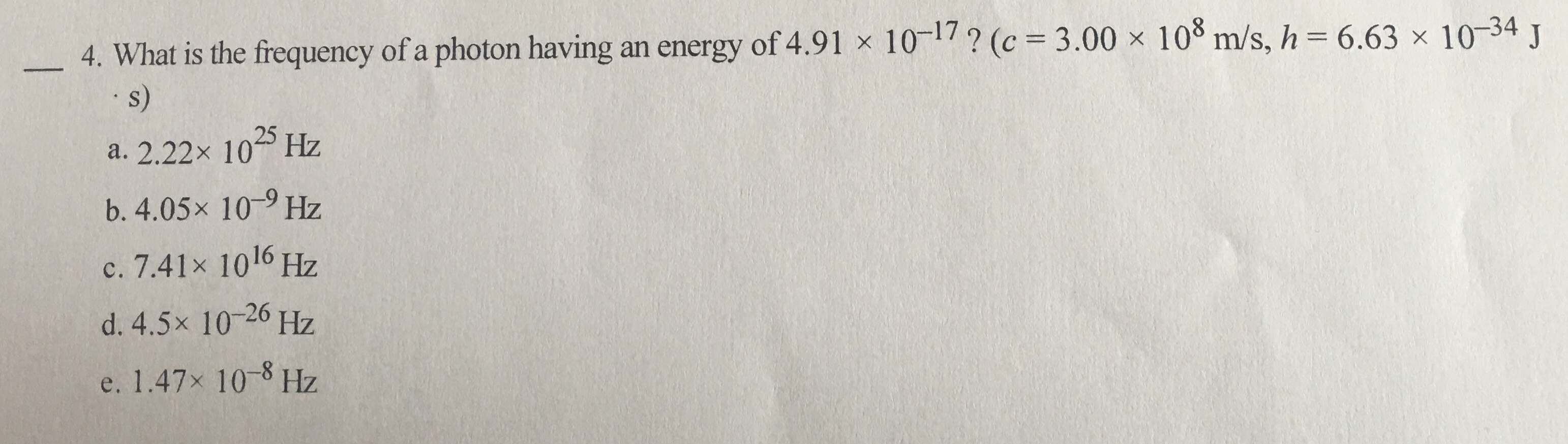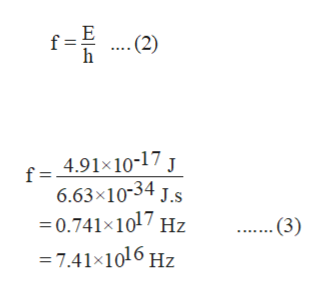4. What is the frequency of a photon having an energy of 4.91 x 101/? (c 3.00 x 103 m/s, hs)6.63 x 1034 J2Hza. 2.22x 10b. 4.05x 10Hzc. 7.41x 1016 Hzd. 4.5x 1020 Hze. 1.47x 10 Hz

Questionhelp_outlineImage Transcriptionclose4. What is the frequency of a photon having an energy of 4.91 x 101/? (c 3.00 x 103 m/s, h s) 6.63 x 1034 J 2 Hz a. 2.22x 10 b. 4.05x 10 Hz c. 7.41x 1016 Hz d. 4.5x 1020 Hz e. 1.47x 10 Hz fullscreen
Step 1

The relation between the energy of a photon and frequency is shown by the equation (1) in which E is the energy of the photon, f is the frequency of photon and h is the Planck’s constant.

Step 2

Rearrange equation (1) for f as shown in equation (2).

Substitute, 4.91×10-17 J for E and 6.63&ti...help_outlineImage Transcriptionclosef (2) h f = 4.91x10-17 J 6.63x10-34 J.s 0.741x1017 Hz 7.41x1016 Hz . (3) fullscreen

Want to see the full answer?

See Solution

Want to see this answer and more?

Our solutions are written by experts, many with advanced degrees, and available 24/7

See Solution
Tagged in

Physical Chemistry